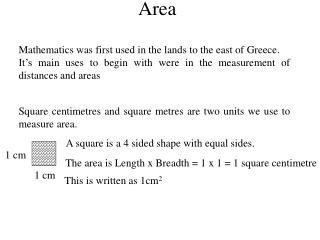DownloadDownload PresentationArea

# Area

Download Presentation## Area

- - - - - - - - - - - - - - - - - - - - - - - - - - - E N D - - - - - - - - - - - - - - - - - - - - - - - - - - -
##### Presentation Transcript

1. Area Mathematics was first used in the lands to the east of Greece. It’s main uses to begin with were in the measurement of distances and areas Square centimetres and square metres are two units we use to measure area. A square is a 4 sided shape with equal sides. 1 cm The area is Length x Breadth = 1 x 1 = 1 square centimetre 1 cm This is written as 1cm2

2. Put the following pictures in order of size, smallest first. (c) (b) (d) (a) (c), (b), (d) and (a)

3. If each shape is formed from square centimetres, which of these shapes have the same area? They all have the same area 9cm2

4. Once again, each square represents 1 square centimetre. What is the area if the shape in the middle? The area = 7 cm2

5. 1 2 3 4 5 1 2 3 4 5 1 2 3 4 5 1 2 3 4 5 1 2 3 4 5 6 7 8 9 10 11 12 13 14 15 16 17 18 19 20 Area of a Rectangle Here is a rectangle that is 5 squares in length and 4 cm in width. One way to find the area is to count the squares. Here we can see that the area is 20cm2. An easier way is to use the formula

6. Page 167 Exercise 2

7. Area of a Right Angled Triangle We can make right angled triangles from the rectangles shown above. It appears that we have cut the rectangles in half. Area of the Right Angled Triangle = ½ Area of the Rectangle

8. (i) First find the area of the surrounding rectangle (ii) Half that answer to get the area of the right angled triangle 7 squares = 7cm 5 squares = 5cm

9. Find the area of the triangle. 8cm 6cm Remember: look for the surrounding rectangle

10. Page 169 Exercise 3

11. 5cm 12cm Area of any Triangle Remember that the height of a triangle is the VERTICAL height 1. Calculate the area of this triangle.

12. 8cm 12cm 2. Calculate the area of this triangle.

13. Page 174 Exercise 4

14. 7 cm 9 cm 1 2 4 cm 12 cm Combining Areas • If a shape is made up of two or more rectangles, to find its area. • Calculate the area of each rectangle • Add the areas together

15. 15 cm 8 cm 1 2 10 cm We can do the same for other shapes made up of rectangles and triangles. Page 176 Exercise 5

16. Area – Extension Exercise The Parallelogram is a four sided shape with parallel sides. If we split the parallelogram into 2 shapes. We then get a right angled triangle. Let us now move the triangle to the other side.

17. Area – Extension Exercise The Parallelogram is a four sided shape with parallel sides. If we split the parallelogram into 2 shapes. Vertical height We then get a right angled triangle. Let us now move the triangle to the other side. base We now get a rectangle. Note the area of the original shape has not changed

18. Area – Extension Exercise The Parallelogram is a four sided shape with parallel sides. Vertical height base

19. The area of this parallelogram is 48cm2. Calculate its height. 8 cm Page 72 Extension Book Question 4 to 10

20. Rhombus and Kite If we imagine a rectangle surrounding the rhombus or kite. The area of the rhombus or kite is half that of the surrounding rectangle

21. Rhombus and Kite If we imagine a rectangle surrounding the rhombus or kite. The area of the rhombus or kite is half that of the surrounding rectangle. Let us consider the length and breadth of the rectangles. If we move these lengths inside the rhombus and kite what will we get?

22. Rhombus and Kite If we imagine a rectangle surrounding the rhombus or kite. The area of the rhombus or kite is half that of the surrounding rectangle. Let us consider the length and breadth of the rectangles. If we move these lengths inside the rhombus and kite what will we get? Let us remove the surrounding rectangles.

23. Rhombus and Kite The length and breadth become the diagonals of the rhombus and kite. A diagonal is a line which joins a vertex to the opposite vertex

24. 5cm Calculate the area of this kite. 12 cm Page 74 Extension Booklet Exercise 2E Question 3 to 7.

25. Trapezium b cm h cm a cm Page 78 Extension Booklet Exercise 6E Question 3 to 4.